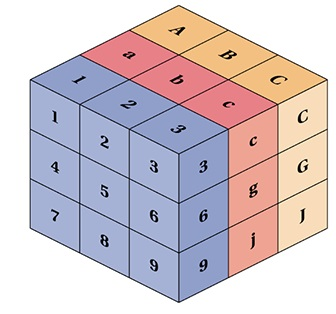# Tensors

A Tensor is a N-dimensional Matrix:

• A Scalar is a 0-dimensional tensor
• A Vector is a 1-dimensional tensor
• A Matrix is a 2-dimensional tensor

A Tensor is a generalization of Vectors and Matrices to higher dimensions.

ScalarVector(s)
1
 1 2 3

 1 2 3

MatrixTensor
 1 2 3 4 5 6

 1 2 3 4 5 6

 4 5 6 1 2 3

## Tensor Ranks

The number of directions a tensor can have in a N-dimensional space, is called the Rank of the tensor.

The rank is denoted R.

A Scalar is a single number.

• It has 0 Axes
• It has a Rank of 0
• It is a 0-dimensional Tensor

A Vector is an array of numbers.

• It has 1 Axis
• It has a Rank of 1
• It is a 1-dimensional Tensor

A Matrix is a 2-dimensional array.

• It has 2 Axis
• It has a Rank of 2
• It is a 2-dimensional Tensor

## Real Tensors

Technically, all of the above are tensors, but when we speak of tensors, we generally speak of matrices with a dimension larger than 2 (R > 2).## Linear Algebra in JavaScript

In linear algebra, the most simple math object is the Scalar:

const scalar = 1;

Another simple math object is the Array:

const array = [ 1, 2, 3 ];

Matrices are 2-dimensional Arrays:

const matrix = [ [1,2],[3,4],[5,6] ];

Vectors can be written as Matrices with only one column:

const vector = [ ,, ];

Vectors can also be written as Arrays:

const vector = [ 1, 2, 3 ];

Tensors are N-dimensional Arrays:

const tensor = [ [1,2,3],[4,5,6],[7,8,9] ];

## JavaScript Tensor Operations

Programming tensor operations in JavaScript, can easily become a spaghetti of loops.

Using a JavaScript library will save you a lot of headache.

One of the most common libraries to use for tensor operations is called tensorflow.js.

const tensorA = tf.tensor([[1, 2], [3, 4], [5, 6]]);
const tensorB = tf.tensor([[1,-1], [2,-2], [3,-3]]);

// Result [ [2, 1], [5, 2], [8, 3] ]

Try it Yourself »

### Tensor Subtraction

const tensorA = tf.tensor([[1, 2], [3, 4], [5, 6]]);
const tensorB = tf.tensor([[1,-1], [2,-2], [3,-3]]);

// Tensor Subtraction
const tensorSub = tensorA.sub(tensorB);

// Result [ [0, 3], [1, 6], [2, 9] ]

Try it Yourself »